# What Is A Reciprocal Function? (3 Key Ideas To Know)

The reciprocal of a number is used often in mathematics to solve algebra problems (with multiplicative inverses).  However, a reciprocal function can also describe a relationship between two variables where one increases as the other decreases.

So, what is a reciprocal function?  The reciprocal function has the form f(x) = 1/x or y = 1/x, and in this case, we can also say that y is inversely proportional to x. The reciprocal function has variations, such as y = k/x (scaling or compression), y = 1/(x-k) (horizontal shift), and y = (1/x) + k (vertical shift).

Of course, the reciprocal function has both a horizontal and a vertical asymptote.  The same is true for any of its variations (scaled or shifted versions).

In this article, we’ll talk about reciprocal functions, what they are, and how to draw them.  We’ll also look at examples of how to work backwards and find the equation of a reciprocal function from a graph or table.

Let’s get started.

## What Is A Reciprocal Function?

The reciprocal function is a basic rational function with the form

• f(x) = 1/x

We can also write the reciprocal function as

• y = 1/x

This is a specific case of inverse variation between the variables y and x.  More generally, we say that y is inversely proportional to x if y = k/x for some real constant k (where k is not zero).

The domain of the reciprocal function is the set of all real numbers, excluding zero.

The reciprocal function is also its own inverse function, since 1/(1/x) = x.

### Why Is It Called A Reciprocal Function?

The function f(x) = 1/x is called the reciprocal function because it takes an input (x-variable) and gives the reciprocal 1/x as the output.

Remember that the reciprocal of any real number x (except x = 0) is 1/x.  For example:

• The reciprocal of 2 is 1/2.
• The reciprocal of 3 is 1/3.
• The reciprocal of 4 is 1/4.
• The reciprocal of -10 is -1/10.
• The reciprocal of 2/5 is 1/(2/5) or 5/2.

### What Does The Reciprocal Function Look Like?

The reciprocal function f(x) = 1/x looks like it has two separate (disconnected) “parts”:

• The “top right” part is to the right of the vertical asymptote x = 0 and above the horizontal asymptote y = 0.
• The “bottom left” part is to the left of the vertical asymptote x = 0 and below the horizontal asymptote y = 0.

You can see the graph of the reciprocal function f(x) = 1/x below: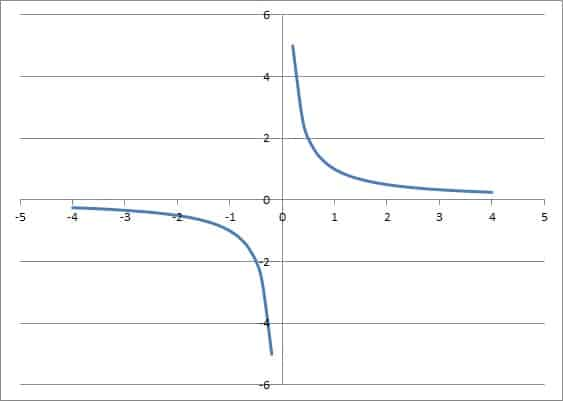This is the graph of the reciprocal function, f(x) = 1/x or y = 1/x. Note that two separate “parts” of the graph: top right and bottom left, which are disconnected. Also note the vertical asymptote at x = 0 and the horizontal asymptote at y = 0. In this case, y is the reciprocal of x, and we can also say that y is inversely proportional to x.

### Shift Or Scale The Reciprocal Function

We can take some variations of the basic reciprocal function f(x) = 1/x to scale or shift the graph as follows:

• f(x) = k/x scales the graph by a factor of k.  If k > 1, then the graph gets “taller” (stretched).  If k < 1, then the graph gets “shorter” (compression).
• f(x) = 1/(x – a) causes a horizontal shift of a units.  If a > 0, then the graph is shifted a units to the right.  If a < 0, then the graph is shifted a units to the left.
• f(x) = (1/x) + b causes a vertical shift of b units.  If b > 0, then the graph is shifted up by b units.  If b < 0, then the graph is shifted down by b units.

Of course, we can combine two or more of these shifts to get any variation we like.  For example, the function g(x) = (2/(x – 1)) + 3 looks like the reciprocal function f(x) = 1/x, but it is shifted 1 unit to the right, 3 units up, and stretched vertically by a factor of 2 (it is “twice as tall” as f(x) = 1/x).

You can see a graph of this scaled and shifted function below.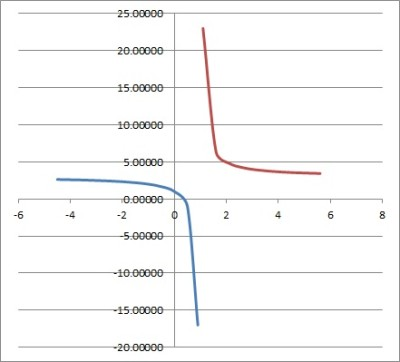This is the graph of the shifted and scaled reciprocal function g(x) = (2/(x – 1)) + 3. It is the function f(x) = 1/x, but scaled by a factor of 2 (twice as tall), with a horizontal shift 1 unit to the right and a vertical shift of 3 units up. Note the vertical asymptote at x = 1 and the horizontal asymptote at y = 3.

### Do Reciprocal Functions Have Symmetry?

The reciprocal function f(x) = 1/x has odd symmetry.  It is an odd function, since:

• f(-x) = 1/(-x)  [by definition of f(x)]
• f(-x) = -(1/x)
• f(-x) = -f(x)  [condition for an odd function]

More generally, any function of the form f(x) = k/x is odd (for k not zero), since:

• f(-x) = k/(-x)  [by definition of f(x)]
• f(-x) = -(k/x)
• f(-x) = -f(x)  [condition for an odd function]

However, a shifted reciprocal function is neither even nor odd.  First, we will look at a horizontal shift f(x) = 1/(x – a):

• f(-x) = 1/(-x – a)  [by definition of f(x)]
• f(-x) = -1/(x + a)

Since f(-x) is not equal to –f(x), and it is not equal to f(x), it is neither even nor odd.

Now, we will look at a vertical shift f(x) = (1/x) + b:

• f(-x) = 1/(-x) + b  [by definition of f(x)]
• f(-x) = -(1/x) + b

Since f(-x) is not equal to –f(x), and it is not equal to f(x), it is neither even nor odd.

### How Do You Draw A Reciprocal Function?

To draw a reciprocal function, it helps to find a few key features first:

• the vertical asymptote (a line x = a that the function does not intersect)
• the horizontal asymptote (a line y = b that the function does not intersect
• a few points (x and y coordinates for both “parts” of the reciprocal function)

For the basic reciprocal function f(x) = 1/x, the vertical asymptote is at x = 0.  For a shifted function f(x) = 1/(x – a), the vertical asymptote is at x = a (basically, whatever value of x gives a zero denominator).

For the basic reciprocal function f(x) = 1/x, the horizontal asymptote is at y = 0.  For a shifted function f(x) = (1/x) + b, the horizontal asymptote is at y = b.

Let’s look at some examples to see how to graph a reciprocal function.

#### Example 1: Graphing The Shifted Reciprocal Function f(x) = 1/(x – 2)

To graph the function f(x) = 1/(x – 2), we need to find the asymptotes and a few points.

The function has a horizontal shift of a = +2, so the vertical asymptote is at x = 2.

The function has no vertical shift, so the horizontal asymptote remains at y = 0.

The following table shows some selected values of x and y for the function:

Using asymptotes and the basic shape of a reciprocal function, we can sketch a graph that goes through the points in the table above.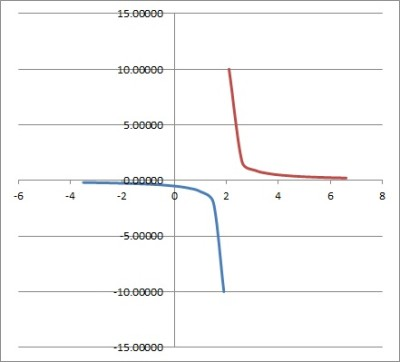This is the graph of the shifted reciprocal function f(x) = 1/(x – 2). It is the function f(x) = 1/x, but with a horizontal shift 2 units to the right. Note the vertical asymptote at x = 2 and the horizontal asymptote at y = 0.

#### Example 2: Graphing The Shifted Reciprocal Function f(x) = (1/x) – 3

To graph the function f(x) = (1/x) – 3, we need to find the asymptotes and a few points.

The function has no horizontal shift, so the vertical asymptote remains at x = 0.

The function has a vertical shift, of b = -3 so the horizontal asymptote is at y = -3.

The following table shows some selected values of x and y for the function:

Using asymptotes and the basic shape of a reciprocal function, we can sketch a graph that goes through the points in the table above.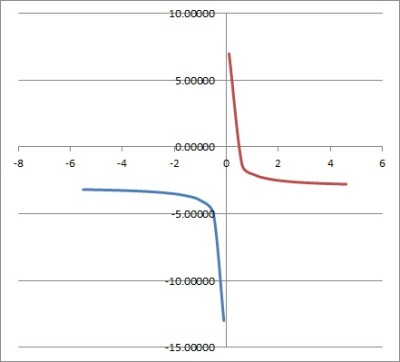This is the graph of the shifted reciprocal function f(x) = (1/x) – 3. It is the function f(x) = 1/x, but with a vertical shift of 3 units down. Note the vertical asymptote at x = 0 and the horizontal asymptote at y = -3.

#### Example 3: Graphing The Scaled & Shifted Reciprocal Function f(x) = (2/(x – 1)) + 5

To graph the function f(x) = (2/(x – 1)) + 5, we need to find the asymptotes and a few points.

The function has a horizontal shift of a = +1, so the vertical asymptote is at x = 1.

The function has a vertical shift of b = +5, so the horizontal asymptote is at y = 5.

Note that the scaling by a factor of 2 (in the numerator) makes the graph “twice as tall” as the basic reciprocal function 1/x.

The following table shows some selected values of x and y for the function:

Using asymptotes and the basic shape of a reciprocal function, we can sketch a graph that goes through the points in the table above.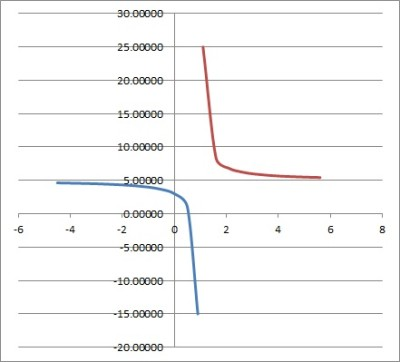This is the graph of the shifted and scaled reciprocal function f(x) = (2/(x – 1)) + 5. It is the function f(x) = 1/x, but scaled by a factor of 2 (twice as tall), with a horizontal shift 1 unit to the right and a vertical shift of 5 units up. Note the vertical asymptote at x = 1 and the horizontal asymptote at y = 5.

### How To Find The Equation Of A Reciprocal Function

We can also work backwards to find the equation of a reciprocal function from either a graph or a table.  Even if we don’t know what scaling or shifting was used, we can figure out the values (by solving equations) if we have enough data points from a table or graph.

Remember that the form of a scaled and shifted reciprocal function is:

• f(x) = (k/(x – a)) + b

which is the function 1/x shifted right a units (left if a < 0), up b units (down if b < 0), and scaled by a factor of k (taller if k > 1, shorter if k < 1).

Let’s look at some examples now.

#### Example 1: How To Find The Equation Of A Reciprocal Function From A Table

Let’s say we have the following table, which comes from a reciprocal function f(x):

Since we have at least 3 points, that is enough to determine the values a, b, and k in our scaled and shifted reciprocal function, which has the form

• f(x) = (k/(x – a)) + b

Substituting the point (-2, -5), we get the equation:

• f(-2) = (k/(-2 – a)) + b
• -5 = (k/(-2 – a)) + b  [Equation A]

Substituting the point (0, 1), we get the equation:

• f(0) = (k/(0 – a)) + b
• 1 = (k/(– a)) + b
• 1 = (-k/a) + b  [Equation B]

Substituting the point (2, -1), we get the equation:

• f(2) = (k/(2 – a)) + b
• -1 = (k/(2 – a)) + b  [Equation C]

Since all 3 equations have a “+b” term, we can cancel by subtracting.

For Equation A minus Equation B:

• -5 – 1 = k/(-2 – a) – (-k/a)  [the +b in both equations cancels]
• -6 = -k/(2 + a) + k/a
• -6a(2 + a) = -ka + k(2 + a)  [multiplied by a(2 + a) on both sides]
• -12a – 6a2 = -ka + 2k + ka  [distribute]
• -12a – 6a2 = 2k  [combine like terms]
• -6a – 3a2 = k  [Equation D]

For Equation C minus Equation B:

• -1 – 1 = (k/(2 – a)) – (-k/a)  [the +b in both equations cancels]
• -2 = (k/(2 – a)) + k/a
• -2a(2 – a) = ka + k(2 – a)  [multiplied by a(2 – a) on both sides]
• -4a + 2a2 = ka + 2k – ka  [distribute]
• -4a + 2a2 = 2k  [combine like terms]
• -2a + a2 = k  [Equation E]

Now, both Equation D and Equation E are solved for k, so we can solve as follows:

• k = k
• -6a – 3a2 = -2a + a2  [from Equation D and Equation E]
• -4a2 – 4a = 0  [combine like terms]
• -4a(a + 1) = 0
• a = 0, -1

If a = 0, then k = 0 by Equation E, but this is impossible (k must not be zero).  So, we must have a = -1.

Then by Equation E, we have:

• -2a + a2 = k  [Equation E]
• -2(-1) + (-1)2 = k  [a = -1]
• 2 + 1 = k
• 3 = k

Since a = -1 and k = 3, then by Equation B we have:

• 1 = (-k/a) + b  [Equation B]
• 1 = (-3/-1) + b  [a = -1, k = 3]
• 1 = 3 + b
• -2 = b

So this reciprocal function has the form f(x) = (k/(x – a)) + b with a = -1, b = -2, and k = 3, which gives us:

• f(x) = (3/(x + 1)) – 2

You can see the graph of this function below.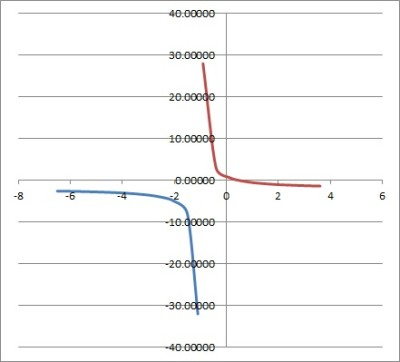This is the graph of the shifted and scaled reciprocal function f(x) = (3/(x + 1)) – 2. It is the function f(x) = 1/x, but scaled by a factor of 3 (three times as tall), with a horizontal shift 1 unit to the left and a vertical shift of 2 units down. Note the vertical asymptote at x = -1 and the horizontal asymptote at y = -2.

#### Example 2: How To Find The Equation Of A Reciprocal Function From A Graph

To find the equation of a reciprocal function from a graph, we can follow the same procedure as in the last example: find 3 points on the graph, write 3 equations, and solve for a, k, and b.

However, we can make things a little easier by just looking at the asymptotes on the graph and then using 1 point to find k.

Consider the graph below: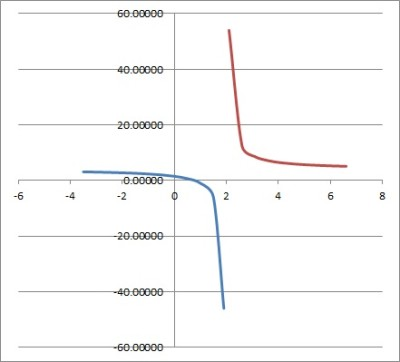This is the graph of the shifted and scaled reciprocal function f(x) = (5/(x – 2)) + 4. It is the function f(x) = 1/x, but scaled by a factor of 5 (five times as tall), with a horizontal shift 2 units to the right and a vertical shift of 4 units up. Note the vertical asymptote at x = 2 and the horizontal asymptote at y = 4.

We can see that this graph has a vertical asymptote at x = 2, meaning that a = 2.

We can also see that this graph has a horizontal asymptote at y = 4, meaning that b = 4.

Now all we need is one point on the graph to find k.  We will use the point (7, 5):

• f(x) = (k/(x – a)) + b  [general form of a scaled & shifted reciprocal function]
• f(x) = (k/(x – 2)) + 4  [a = 2 and b = 4, based on the asymptotes]
• 5 = (k/(7 – 2)) + 4  [from the point (7, 5) on the graph]
• 1 = k/(5)
• 5 = k

So this reciprocal function has the form f(x) = (k/(x – a)) + b with a = 3, b = 4, and k = 5, which gives us:

• f(x) = (5/(x – 2)) + 4

## Conclusion

Now you know what the reciprocal function is and what its variations look like.  You also know how to graph them and how to find the equation of a reciprocal function from a table or graph.